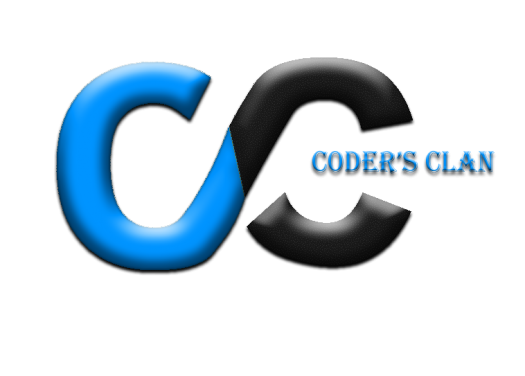# Coder's Clan(Developer Recruit - Round 1)

30 Questions | Total Attempts: 83SettingsCreate your own Quiz• 1.
What is the correct value to return to the operating system upon successful completion of a program in c?
• A.

0

• B.

1

• C.

-1

• D.

Program do not return a value

• 2.
What will be the output after execution of following code?#include<stdio.h>#include<stdlib.h>int main(){char ch;int i;ch='G';i=ch-'A';printf("%d",i);}
• A.

8

• B.

7

• C.

6

• D.

5

• E.

Error

• 3.
What is the output of following code in c?#include#includeint main(){int i=0678;printf("%d",i);return 0;}
• A.

0678

• B.

678

• C.

Compilation error

• D.

Runtime error

• 4.
"My salary was increased 15%"Select the statement which will EXACTLY reproduce above line.
• A.

Printf("My salary was increased 15%");

• B.

Printf("My salary was increased 15\%");

• C.

Printf("My salary was increased '15%' ");

• D.

Printf("My salary was increased 15%%");

• 5.
What is the output of following?#include <stdio.h>#include <stdlib.h>int main() {     char str[] = "Smaller";    int a = 100;    printf(a > 10 ? "Greater" : "%s", str);    return 0;}
• A.

Greater

• B.

Smaller

• C.

100

• D.

Compilation error

• 6.
How many times below for loop will run?#include<stdio.h>int main(){int i=0;for(;;){printf("%d",i++);}return 0;}
• A.

0 times

• B.

Infinite times

• C.

1 time

• D.

Generates error

• 7.
What is the output of following?#include#includeint main(){int i=1;printf("%d%d%d",i++,i,++i);return 0;}
• A.

124

• B.

Compiler dependent

• C.

223

• D.

233

• 8.
Guess the output. #include<stdio.h>int main(){    int a = 100, b = 200, c = 300;    if(!a >= 500)        b = 300;    c = 400;    printf("%d,%d,%d",a, b, c);    return 0;}
• A.

100,200,300

• B.

100,200,400

• C.

100,300,400

• D.

100,300,300

• 9.
What is the output of following? #include<stdio.h> void main(){   int i = 1;   while(i++<=5);       printf("%d ",i);}
• A.

7

• B.

6

• C.

5

• D.

4

• 10.
Linker generate _________ file?
• A.

Object code

• B.

Assembly code

• C.

Executable code

• D.

None of above

• 11.
Which of the following is not a keyword in java?
• A.

Static

• B.

Boolean

• C.

Void

• D.

Private

• 12.
What is the size of byte variable?
• A.

8 bit

• B.

16 bit

• C.

32 bit

• D.

64 bit

• 13.
What is static block?
• A.

It is used to create syncronized code.

• B.

There is no such block.

• C.

It is used to initialize the static data member and It is excuted before main method at the time of class loading.

• D.

None of the above.

• 14.
What happens when thread's sleep() method is called?
• A.

• B.

Thread returns to the waiting state.

• C.

• D.

None of the above.

• 15.
Which method of the Runnable interface that must be implemented by all threads?
• A.

Run()

• B.

Start()

• C.

Sleep()

• D.

Wait()

• 16.
Specify the directory name where the XML layout files are stored in android.
• A.

/assets

• B.

/src

• C.

/values

• D.

None of the Above

• 17.
Can We inflate multiple layout or fragment on the same screen?
• A.

True

• B.

False

• 18.
Which Build System/Tool is used to Build APK in Android Studio?
• A.

• B.

Ant

• C.

Maven

• D.

Ansible

• 19.
What is wrap_content Layout parameter.
• A.

View wants to be as big as its parent (minus padding)

• B.

View wants to be just big enough to enclose its content (plus padding)

• C.

Both (A) and (B)

• D.

None of the Above

• 20.
Which database is Serverless and mostly used for Stand-alone Devices?
• A.

MySql

• B.

SQLite

• C.

MongoDB

• D.

None of the Above

• 21.
Who is known as father of PHP ?
• A.

Charles Reym

• B.

Rasmus Lerdraf

• C.

Prof. Thales

• D.

Louis Pasture

• 22.
How a variable declared in PHP ?
• A.

\$varname=\$value;

• B.

Varname=value;

• C.

\$varname=value;

• D.

None of These

• 23.
What will the following script output?<?php\$array = array (1, 2, 3, 5, 8, 13, 21, 34, 55);\$sum = 0;for (\$i = 0; \$i < 5; \$i++) {\$sum += \$array[\$array[\$i]];}echo \$sum;?>
• A.

78

• B.

19

• C.

Null

• D.

5

• 24.
In PHP in order to access MySQL database you will use:
• A.

Mysqlconnect() function

• B.

Mysql-connect() function

• C.

Mysql_connect() function

• D.

Sql_connect() function

• 25.
The ............. statement is used to delete a table.
• A.

Drop Table

• B.

Delete Table

• C.

Del table

• D.

Remove Table

Related TopicsBack to top
×

Wait!
Here's an interesting quiz for you.